• ## MATLAB中ode23函数，龙格库塔函数

万次阅读 多人点赞 2017-04-25 16:43:38
今天说一说MATLAB中ode23函数的原理，在网上看了好多，但是不知道是怎么计算的，就知道是那么用的，但是最后结果咋回事不知道，今天来讲一讲是怎么计算的。 首先来个程序：function f=eg6fun(t,y) f=-y^3-2; end...
        今天说一说MATLAB中ode23函数的原理，在网上看了好多，但是不知道是怎么计算的，就知道是那么用的，但是最后结果咋回事不知道，今天来讲一讲是怎么计算的。
首先来个程序：
function f=eg6fun(t,y)
f=-y^3-2;
end上面是我定义的一个函数，看着挺简单的哈！不多说了。

[t,y]=ode23(@eg6fun,[0,30],1);这句话是我用ode23调用的语句，先说一下，这里eg6fun是我上面函数的名称，也就是ode23主要计算的就是这个函数的微分。[0,30]为t的范围，这里t没有什么太大的作用，只是为了计算步长用的，之后我把运行后的t和y数据粘在这里，我们发现，在MATLAB中步长并不是固定的。这里应该是用一个什么函数求得，我没查，感兴趣的自己查一下。1为y的初值，也就是我们常常说的y0。

先粘上实验结果，我们在分析怎么来的：
下面的是t的值，这里MATLAB将t在[0,30]区间分成了67份，我这里只粘了一部分：
0 0.0266666666666667 0.0974376132058027 0.178185989598692 0.270160382755732 下面是y的结果，y最后也是一个[1,67]的矩阵：
1 0.922959859735161 0.740501273051361 0.556672216994644 0.363414446549133
下面我们来说是怎么计算的吧！看下面的图，这个是我在数值分析书上照的，其实ode23就是龙格库塔函数的应用，而龙格库塔函数就是根据欧拉法得来的，看下图：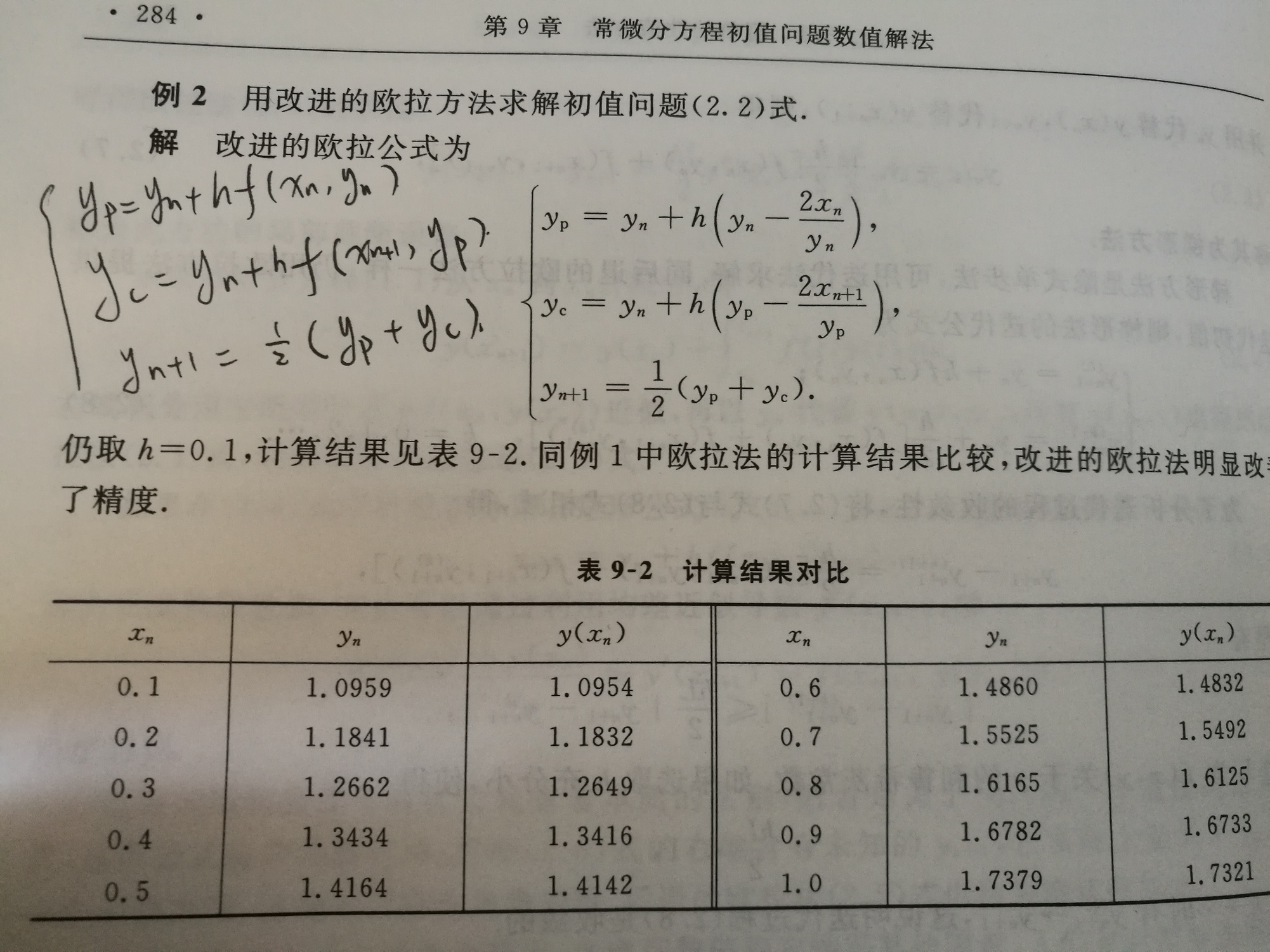上面图片中有三个公式，第一个公式h后面括号中的内容就是要求积分的函数，就是我们程序中的eg6fun。那么就好办了，把图中公式中的括号中的内容换成我们的公式也就是

-y^3-2
然后计算就好了。这里h为步长，也就是我们程序中t的步长，我们可以看到第一次t为0.0266666666666667，而下一次的步长为0.0974376132058027-0.0266666666666667，只要这么一步一步计算就好了。
（这里看图中黑色笔手写的公式）
这里计算一步来表示计算的大概过程：
例如： (1)计算Yp=y1+h * (-y1^3 - 2) = 1 - 0.027*3 = 0.919
(2)       Yc=y1+h * (-Yp^3 - 2) = 1 - 0.027*(0.919^3 - 2) = 0.925
(3)        Y(n+1) = 1/2 * (Yp + Yc) = 1/2 * (0.919 + 0.925) = 0.922
因为这里我们保留精度为3位小数，可能计算的有些误差。还有一点需要注意，龙格库塔函数是对欧拉方法进行的改进，其实龙格库塔函数的精度要比欧拉方法更高。因此这里计算有些许误差。但是大概的过程就是这样的。

上面的内容是之前写的，讲解的是欧拉算法计算微分的过程，其实龙格-库塔方法后来在书中看到，下面介绍一下龙格库塔方法：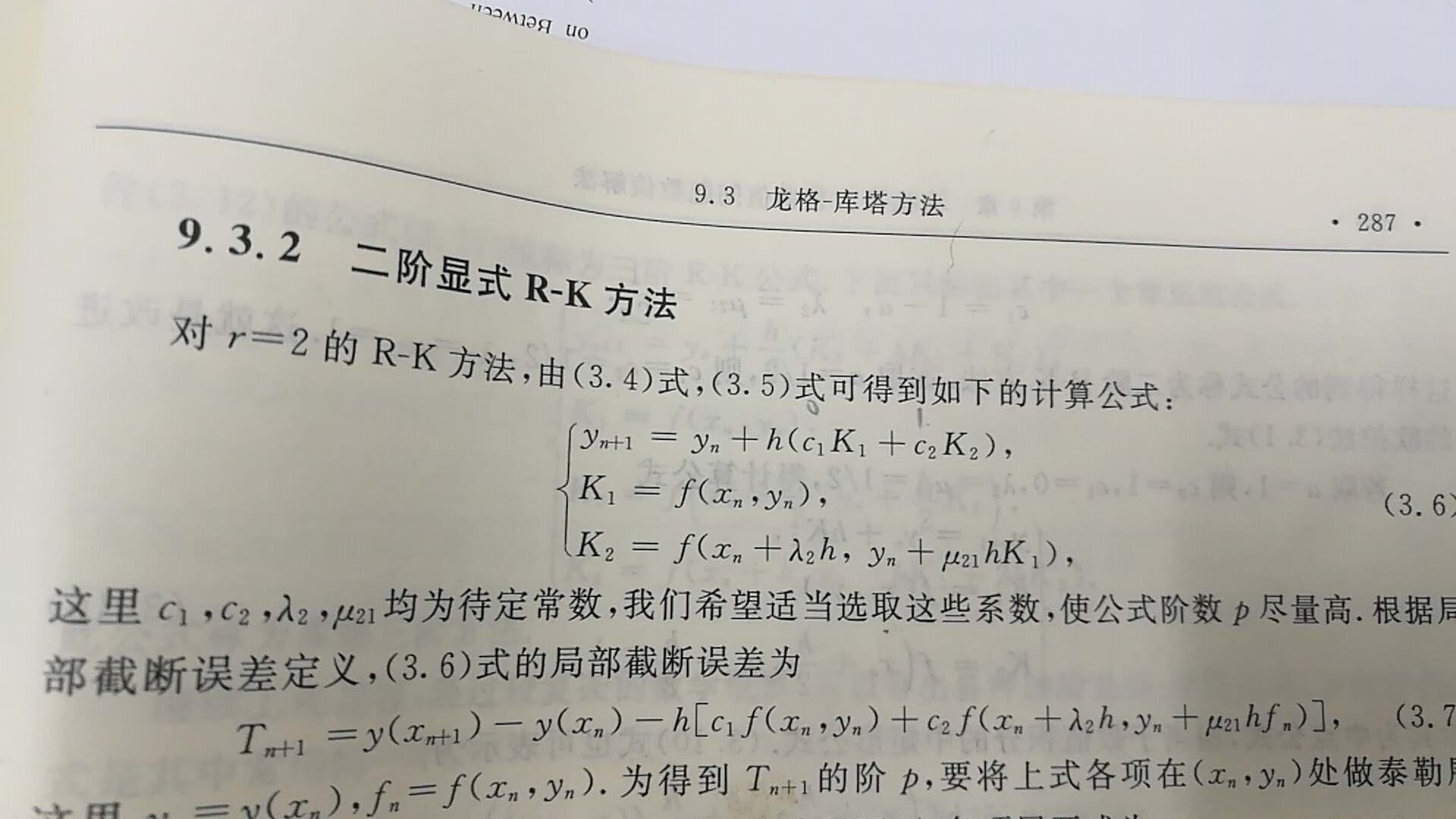MATLAB中的ode23就是用的二阶的龙格库塔方法，就是图中3.6的三个公式，这里h为步长，上面给出的t，c1和c2是系数，这个系数取值不是固定的，MATLAB中是啥我也不是确定，但是书中最后给的是c1=0，c2=1，λ2和μ21取值1/2。这样一来，计算一波：y1=1;求y2，将y1带入公式中的yn，这里没有x，所以有x的项可以忽略
k1=-3；
k2=f(1-(1/2)*0.0267*3)=f(0.96)=-2.88
y2=1-0.0267*2.88=0.923
y2求出，其余的过程都是这样求得。ode45是四阶龙格库塔函数，下图为4阶求法，这里不再做介绍：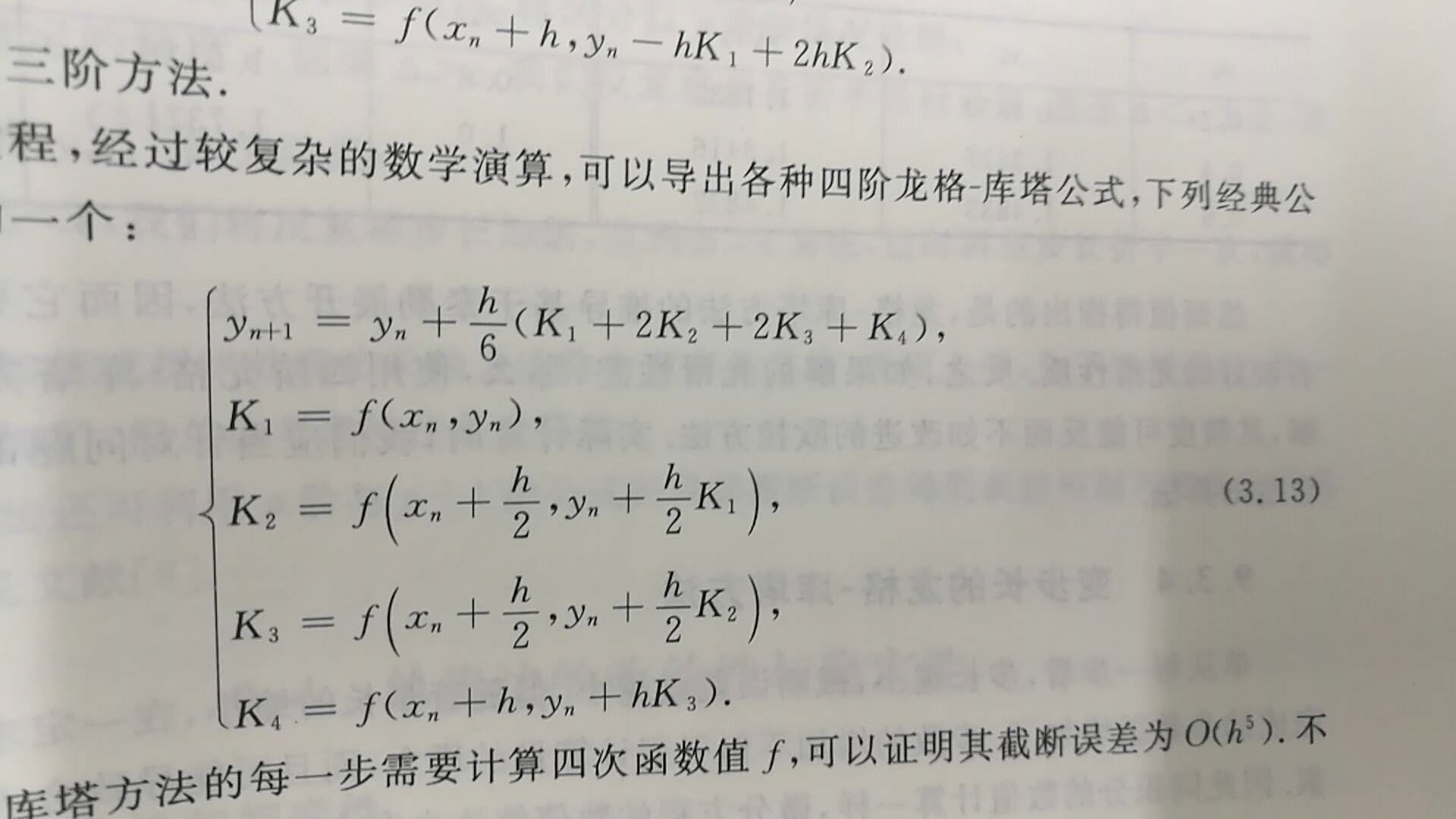到此MATLAB中ode23的计算方法已经讲解完了，当然，ode45跟这个应该类似，就是ode45比ode23更精确一点，在MATLAB中，如果我们用ode45会发现，t在[0,30]间分成了167份，很明显精度提高了。其实MATLAB中有好多的函数都是用到了数值分析中的内容，而数值分析就是用我们的笨方法来计算数值的一种工具，这是我自己定义的哈，通过减少误差来使计算出来的数据更准确。

展开全文MATLAB 微分方程
• 本文主要介绍matlab中求解常微分方程（组）的dsolve和ode系列函数，并通过例子加深读者的理解。 一、符号介绍  D: 微分符号；D2表示二阶微分，D3表示三阶微分，以此类推。 二、函数功能介绍及例程 1、dsolve ...
本文主要介绍matlab中求解常微分方程（组）的dsolve和ode系列函数，并通过例子加深读者的理解。
一、符号介绍
D: 微分符号；D2表示二阶微分，D3表示三阶微分，以此类推。
二、函数功能介绍及例程
1、dsolve 函数
dsolve函数用于求常微分方程组的精确解，也称为常微分方程的符号解。如果没有初始条件或边界条件，则求出通解；如果有，则求出特解。
1)函数格式
Y = dsolve(‘eq1,eq2,…’ , ’cond1,cond2,…’ , ’Name’)
其中，‘eq1,eq2,…’:表示微分方程或微分方程组;
’cond1,cond2,…’:表示初始条件或边界条件;
‘Name’:表示变量。没有指定变量时，matlab默认的变量为t；
2)例程
例1.1(dsolve 求解微分方程)
求解微分方程：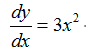在命令行输入: dsolve('Dy=3*x^2','x') ,摁下enter键后输出运行结果。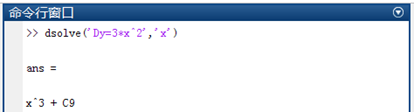例1.2（加上初始条件）
求解微分方程：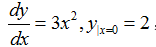只需要在命令行添加初始条件即可，此时求出的即为方程的特解。可以看到上例中的C9变为了2。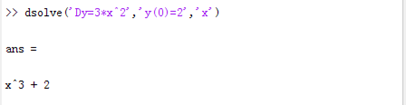例2(dsolve 求解微分方程组)
求解微分方程组：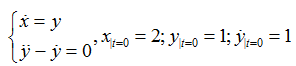由于x,y均为t的导数，所以不需要在末尾添加’t’。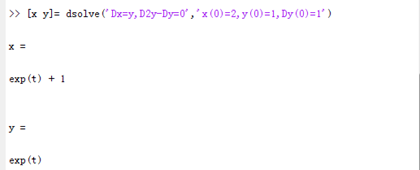2、ode函数
在上文中我们介绍了dsolve函数。但有大量的常微分方程，虽然从理论上讲，其解是存在的，但我们却无法求出其解析解，此时，我们需要寻求方程的数值解。
怎么理解数值求解呢？数值分析是一门专门的学科，在此不过多介绍。我主要想通过一个简单的例子来向大家阐述数值求解的思想。
比如，求解微分方程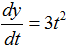。我们就可以转化为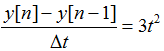，那么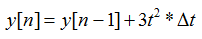。因此，我们可以通过迭代的方式来求解y。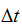即可理解为步长
ode是Matlab专门用于解微分方程的功能函数。该求解器有变步长（variable-step）和定步长（fixed-step）两种类型。不同类型有着不同的求解器，具体说明如下图。
非刚性ode求解命令  求解器solver  功能  说明  ode45  一步算法：4、5阶龙格库塔方程：累计截断误差(Δx)^5  大部分尝试的首选算法  ode23  一步算法：2、3阶龙格库塔方程：累计截断误差(Δx)^3  适用于精度较低的情形  ode113  多步算法：Adams  计算时间比ode45短  刚性ode求解命令  ode23t  梯形算法  适度刚性情形  ode15s  多步法：Gear’s反向数值微分：精度中等  若ode45失效时，可以尝试使用  ode23s  一步法：2阶Rosebrock算法：精度低  当精度较低时，计算时间比ode15s短  ode23tb  梯形算法：精度低  当精度较低时，计算时间比ode15s短
其中，ode45求解器属于变步长的一种，采用Runge-Kutta算法；其他采用相同算法的变步长求解器还有ode23。ode45表示采用四阶-五阶Runge-Kutta算法，它用4阶方法提供候选解，5阶方法控制误差，是一种自适应步长（变步长）的常微分方程数值解法，其整体截断误差为(Δx)^5。解决的是Nonstiff(非刚性)常微分方程。
ode45是解决数值解问题的首选方法，若长时间没结果，应该就是刚性的，可换用ode15s试试。
下面将以ode45为例具体介绍函数的使用方法。
1)函数格式
[T,Y] = ode45(‘odefun’,tspan,y0)
[T,Y] = ode45(‘odefun’,tspan,y0,options)
[T,Y,TE,YE,IE] = ode45(‘odefun’,tspan,y0,options)
sol = ode45(‘odefun’,[t0 tf],y0...)
其中: odefun是函数句柄,可以是函数文件名,匿名函数句柄或内联函数名；
tspan 是求解区间 [t0 tf]，或者一系列散点[t0,t1,...,tf]；
y0 是初始值向量
T 返回列向量的时间点
Y 返回对应T的求解列向量
options 是求解参数设置,可以用odeset在计算前设定误差,输出参数,事件等
TE 事件发生时间
YE 事件发生时之答案
IE 事件函数消失时之指针i
2)微分方程标准化
利用ode45求解高阶微分方程时，需要做变量替换。下面说明替换的基本思路。
微分方程为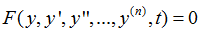初始条件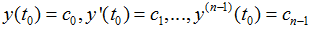首先做变量替换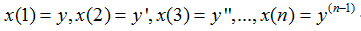原微分方程可以转换为下面的微分方程组的格式: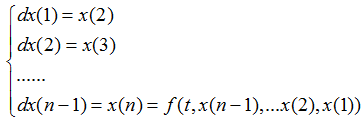下面就可以利用转换好的微分方程组来编写odefun函数。
3）例程
例3.1（编写odefun函数）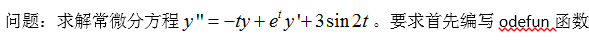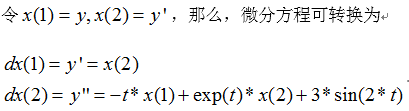在matlab中新建脚本文件，编写函数如下：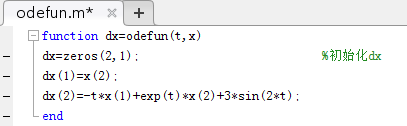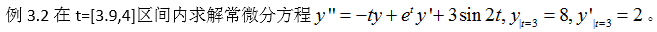本例中只需在例3.1的基础上编写主函数，加上求解区间和边值条件即可。需要注意的是，ode45的运行结果以列向量形式给出。因此在本例中，x的第一列为y，第二列为y’。如果遇到变量不是列向量形式的，可以考虑利用reshape函数做矩阵变换。
则，plot(t,x(:,1))画出来的是x的第一列数据，即为y；
plot(t,x(:,2))画出来的是x的第二列数据，即为y’；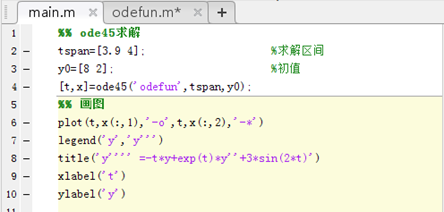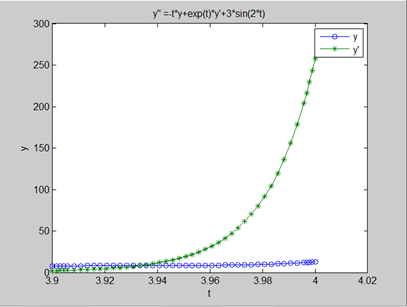三、总结
在使用matlab中，如遇到命令格式记不清楚等情况，建议直接在命令行输入指令’help+函数名称’，如，在matlab命令窗口输入help dsolve后，显示如下：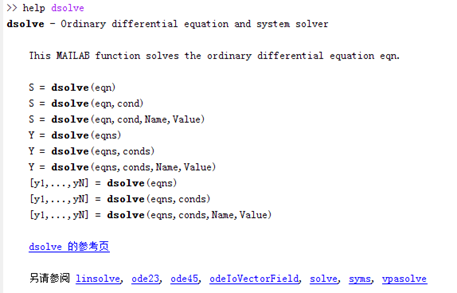参考：
1、https://jingyan.baidu.com/article/e52e36154448e940c60c51aa.html
3、https://wenku.baidu.com/view/45a0a0b54b73f242326c5f7f.html


展开全文• 原文地址：ode45 函数传自定义参数用法及定步长ode5解算函数">matlab ode45 函数传自定义参数用法及定步长ode5解算函数作者：jlxiaohuo要用的时候总是忘记，这回给把它写在这里！   %%程序1 arg1 = 2; arg2 = 1;...


原文地址：matlab ode45 函数传自定义参数用法及定步长ode5解算函数
作者：jlxiaohuo

要用的时候总是忘记，这回给把它写在这里！

%%程序1
arg1 = 2; arg2 = 1; [T,Y] = ode45('vdp1000',[0 10],[2 0], [], arg1, arg2); plot(T,Y(:,1),'-o');

%%程序2
function dy = vdp1000(t, y, flag, arg1, arg2) dy =zeros(2,1);    %a column vector dy(1) = y(2); dy(2) = arg1*(arg2 - y(1)^2)*y(2) - y(1);

%%ode5
function Y = ode5(odefun,tspan,y0,varargin)
%ODE5 Solve differential equations with a non-adaptive method oforder 5.
% Y = ODE5(ODEFUN,TSPAN,Y0) with TSPAN = [T1, T2, T3, ... TN]integrates % the system of differential equations y' = f(t,y) by stepping fromT0 to % T1 to TN. Function ODEFUN(T,Y) must return f(t,y) in a columnvector. % The vector Y0 is the initial conditions at T0. Each row in thesolution % array Y corresponds to a time specified in TSPAN. % % Y = ODE5(ODEFUN,TSPAN,Y0,P1,P2...) passes the additionalparameters % P1,P2... to the derivative function asODEFUN(T,Y,P1,P2...). % This is a non-adaptive solver. The step sequence is determined byTSPAN % but the derivative function ODEFUN is evaluated multiple timesper step. % The solver implements the Dormand-Prince method of order 5 in ageneral % framework of explicit Runge-Kutta methods. % % Example % tspan = 0:0.1:20; % y = ode5(@vdp1,tspan,[2 0]); % plot(tspan,y(:,1)); % solves the system y' = vdp1(t,y) with a constant step size of0.1, % and plots the first component of the solution.
if ~isnumeric(tspan)         error('TSPANshould be a vector of integration steps.');     end
if ~isnumeric(y0)         error('Y0should be a vector of initial conditions.');     end
h = diff(tspan);
if any(sign(h(1))*h <= 0)        error('Entries of TSPAN are not in order.')     end
try         f0 =feval_r(odefun,tspan(1),y0,varargin{:});     catch         msg =['Unable to evaluate the ODEFUN at t0,y0. ',lasterr];        error(msg);     end
y0 = y0(:); % Make a column vector.
if ~isequal(size(y0),size(f0))        error('Inconsistent sizes of Y0 and f(t0,y0).');     end
neq = length(y0);
N = length(tspan);
Y = zeros(neq,N);
% Method coefficients -- Butcher's tableau
%
% C | A
% --+---
% | B
C = [1/5; 3/10; 4/5; 8/9; 1];
A = [ 1/5, 0, 0, 0, 0     3/40, 9/40, 0, 0, 0
44/45 -56/15, 32/9, 0, 0
19372/6561, -25360/2187, 64448/6561, -212/729, 0
9017/3168, -355/33, 46732/5247, 49/176, -5103/18656];
B = [35/384, 0, 500/1113, 125/192, -2187/6784, 11/84];
% More convenient storage
A = A.';
B = B(:);
nstages = length(B);
F = zeros(neq,nstages);
Y(:,1) = y0;
for i = 2:N         ti =tspan(i-1);         hi =h(i-1);         yi =Y(:,i-1);         % Generalexplicit Runge-Kutta framework         F(:,1) =feval_r(odefun,ti,yi,varargin{:});         for stage =2:nstages                tstage = ti + C(stage-1)*hi;                ystage = yi + F(:,1:stage-1)*(hi*A(1:stage-1,stage-1));                F(:,stage) = feval_r(odefun,tstage,ystage,varargin{:});             end         Y(:,i) = yi+ F*(hi*B);     end
Y = Y.';


展开全文• ode45函数无法求出解析解，dsolve可以求出解析解(若有)，但是速度较慢. 1.      ode45函数 ①求一阶常微分方程的初值问题 [t,y] = ode45(@(t,y)y-2*t/y,[0,4],1); plot(t,y); ...


ode45函数无法求出解析解，dsolve可以求出解析解(若有)，但是速度较慢.
1.      ode45函数
①求一阶常微分方程的初值问题
[t,y] = ode45(@(t,y)y-2*t/y,[0,4],1);
plot(t,y);
求解 y’ – y + 2*t / y且初值y(0) = 1的常微分方程初值问题，返回自变量和函数的若干个值.
若不写返回值，则会自动作出函数随自变量的变化图像.
ode45(@(t,y)y-2*t/y,[0,4],1);

②求解一阶微分方程组
x’ = -x^3-y,x(0)=1
y’ = x-y^3,y(0)=0.5.
自变量为t,且0<t<30.
求解过程如下.
第一步，在M函数文件中将函数x和函数y写成向量形式.
function f = fun(t,x);
f(1) = -x(1)^3 – x(2);
f(2) = x(1) – x(2)^3;
f = f(:);%确保f为列向量.
第二步，在M脚本文件中求解.
[t,x] = ode45(@fun,[0,30],[1;0.5]);
subplot(1,2,1);plot(t,x(:,1),t,x(:,2),':');xlabel('t');ylabel('x/y');%作x和y随t变化图
subplot(1,2,2);plot(x(:,1),x(:,2));xlabel('x');ylabel('y');%作x和y的相位图
第三步，在命令窗口运行M脚本文件中的代码.

③求解高阶常微分方程组
将高阶常微分方程组通过变量替换转化为一阶的常微分方程组，然后用ode45求解.

2.      dsolve函数
①求解析解
y’ = a*x + b;
s = dsolve('D2y=a*y+b*x','x');
D2y用以表示y的二阶导数，默认是以t为自变量的，所以最好指明自变量为x.

②初值问题
y’ = y – 2*t / y , y(0) = 1;
s = dsolve('Dy == y - 2*t / y','y(0) ==1');

③边值问题
x*y’’ – 3*y’ = x^2 , y(1) = 0 , y(5) = 0;
s = dsolve('x*D2y - 3*Dy ==x^2','y(1)=0','y(5) == 0','x');
函数最后一个参数指明自变量为x.

④高阶方程
求解y’’ = cos(2x) – y , y(0) = 1 , y’(0) = 0;
s=dsolve('D2y == cos(2*x) - y','y(0) =1','Dy(0) = 0','x');
simplify(s);

⑤方程组问题
f’ = f + g , g’ = -f + g,f(0) = 1, g(0) =2;
[f,g]= dsolve('Df == f + g','Dg = -f + g','f(0)==1','g(0) == 2','x');


展开全文• 有问题可以给我发邮件，邮箱地址在上传的资源包里。matlab
• 这是一个嵌套函数的解决方案：function [ dealfunchandle ] = dealwithit( arrayfunc )% Takes as input an event function of (t,z) that returns a 3 array (in same order as event syntax).function ...
• 本文将分为三篇介绍，前两篇分别介绍Isqucurvefit的基本用法和ode45函数的常见和扩展用法。 现在你看到的是第三篇，这篇将把Isqucurve函数ode45函数结合起来，同时完成求解常微分方程和曲线优化拟合两件事。 ...matlab 数学建模
• ## ode中函数参数传递

千次阅读 2018-06-17 15:30:29
转自：http://ma-yue.net/blog/archives/89在用odesolver(ode45, ode15s, …)来解微分方程的时候，最基本的用法是：[t, y] = odesolver(odefun, tspan, y0);这里的odefun是待求的微分方程。那么odefun中一般会含有多...
• 4阶5级龙格库塔法用于解一阶微分方程(组)，对于高阶微分方程，可以将其转换为一阶微分方程组求解。原程序由John.H....该程序精度比matlab自带的ode45更高。rkf45.m:function [Rt Rx]=rkf45(f,tspan,ya,m,tol)%...
• orbitode.m 中嵌套的事件函数将搜索两个事件。一个事件查找距离起点最远的点，另一个事件查找宇宙飞船返回到起点的点。即使积分器使用的步长并非通过事件位置确定，也会准确定位事件。在此示例中，指定过零方向的...
• 本人用ode15s算一个偏微分方程组，该方程组中有两个参数要通过另外一个隐函数方程组求解，不知道怎么调用，ode15s求该解偏微分方程组代码([t,y]=ode15s(@fangcheng,tspan,y0,options);)如下：function dydt=...
• 要用的时候总是忘记，这回给把它写在这里！...[T,Y] = ode45('vdp1000',[0 10],[2 0], [], arg1, arg2); plot(T,Y(:,1),'-o');   %%程序2 function dy = vdp1000(t, y, flag, arg1, arg2)matlab
• 1.来源 在CUHK学习advanced robotics时做hopping robot仿真时多次使用...2.ODE45函数调用 [t,x,te,xe，ie] = ode45(@(t,x) myStanceODEFct(t,x,modelPara,Tau(t,x)), t_span, x0, option); [t,y,te,ye,ie] = ode...event
• 如何使用matlab中的ode45函数进行仿真，详细讲解，并有多个实例解说！
• 这个怎么用MATLAB的ode函数来弄呀，我看其他教程都是只有一个方程，；两个方程怎么弄呀，请教一下！matlab
• 本文主要介绍matlab中求解常微分方程（组）的dsolve和ode系列函数，并通过例子加深读者的理解 matlab求解常微分方程（组）---dsolve、ode系列函数详解（含例程）
•matlab
• ode15s 求解器是适用于大多数刚性问题的首选求解器。但是，对于特定类型的问题，其他刚性求解器可能更高效。本示例使用所有四个刚性 ...这些值使该问题足够刚性，从而使 ode45 和 ode23 需要对该方程进行积分。此外...
• matlab中节点15s函数代码本地化遇见网络 用于 G. Menon 和 J. Krishnan, Spatial Localization meet Biomolecular Networks, 2021 的 Matlab 代码示例 提供的所有文件都包含 Matlab 函数。 文件 nfxlap 和 perlap ...
• 最近因科研工作需求，要用到Runge-Kutta Method解二阶ODE，自己也懒得造轮子了，所以干脆就直接使用Matlab的内置函数ode45来解决（该内置函数基于Explicit Runge-Kutta Method）。于此处记录一下ode45的一些比较有效...
• MATLAB中函数ODE45的使用及详解。帮助深入认识ODE45
• <p style="text-align:center"><img alt="" height="1080" src="https://img-ask.csdnimg.cn/upload/1606728177964.png" width="1920" /></p> <p style="text-align:center"><img alt="" height="1080" src... ...  </p>开发语言 Golang...